# OAE Mathematics: Trigonometric Functions Chapter Exam

Exam Instructions:

Choose your answers to the questions and click 'Next' to see the next set of questions. You can skip questions if you would like and come back to them later with the yellow "Go To First Skipped Question" button. When you have completed the practice exam, a green submit button will appear. Click it to see your results. Good luck!

### Page 1

#### Question 1 1. What is z in terms of x and y?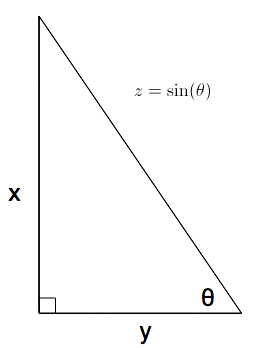#### Question 4 4. In the pictured triangle, side a is 32, side b is 24, and side c is 37. What is the approximate measure of ∠C? When calculating the inverse cosine, round the initial value to the nearest whole number.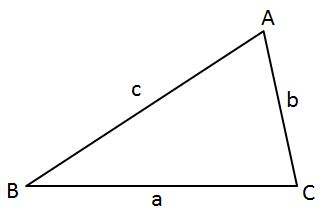#### Question 5 5. In the pictured triangle, ∠A is 58 degrees and ∠C is 63 degrees. If side a is 84 units long, approximately how long is side c?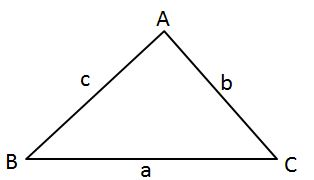### Page 2

#### Question 6 6. What is x?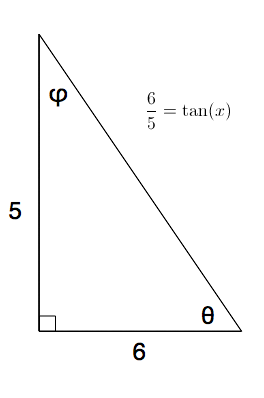### Page 3

#### Question 14 14. What is x?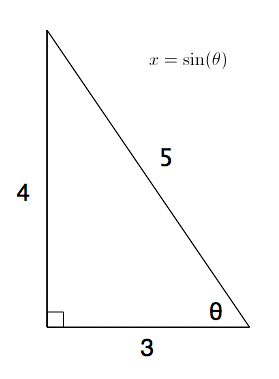#### Question 15 15. In the pictured triangle, side b is 12 and side c is 16. If ∠A is 84 degrees, then approximately how long is side a? Round the value of the cosine to the nearest whole number.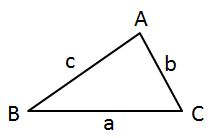### Page 4

#### Question 16 16. In the pictured triangle, ∠A is 62 degrees and ∠C is 86 degrees. If side a is 112 units long, approximately how long is side c?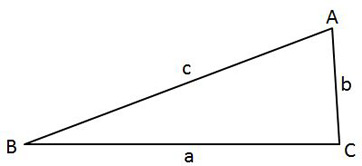#### Question 19 19. The lines a and b are parallel lines of length 5 and 2. The lines d and e are parallel to one another, and both are perpendicular to a and b. What is the length of c?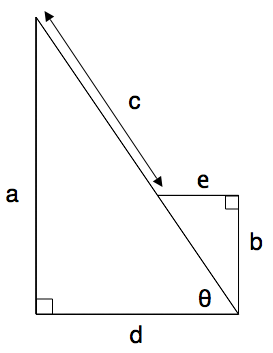#### Question 20 20. In the pictured triangle, ∠A is 98 degrees and ∠B is 12 degrees. If side a is 84 units long, approximately how long is side b?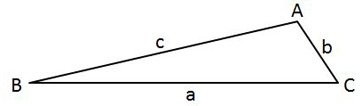### Page 5

#### Question 21 21. In the pictured triangle, ∠A is 81 degrees and ∠B is 67 degrees. If side a is 34 units long, approximately how long is side b?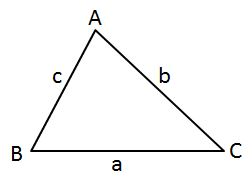#### Question 22 22. What is x?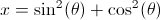#### Question 23 23. What is z in terms of x?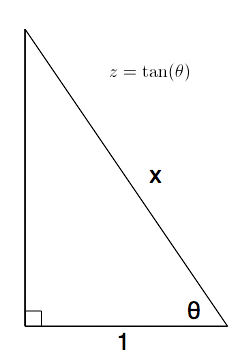#### Question 24 24. In the pictured triangle, ∠A is 137 degrees and ∠B is 28 degrees. If side b is 71 units long, approximately how long is side a?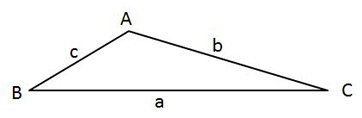### Page 6

#### Question 28 28. In the pictured triangle, what ratio represents the cosine of theta?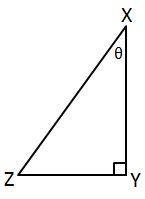#### OAE Mathematics: Trigonometric Functions Chapter Exam Instructions

Choose your answers to the questions and click 'Next' to see the next set of questions. You can skip questions if you would like and come back to them later with the yellow "Go To First Skipped Question" button. When you have completed the practice exam, a green submit button will appear. Click it to see your results. Good luck!

Support